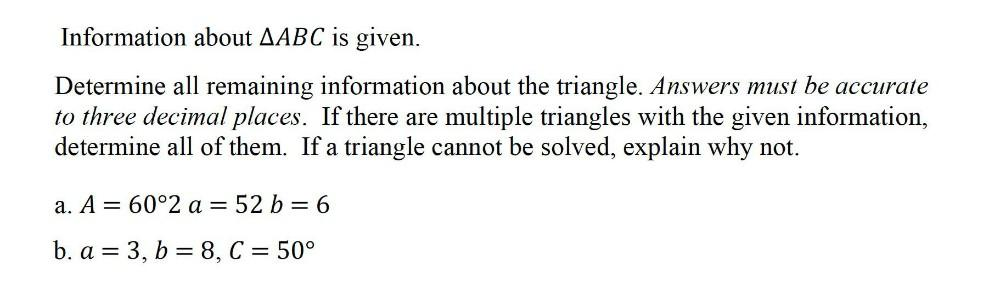Question:

# Information about △ABC is given. Determine all remaining information about the triangle. Answers must be accurate to three decimal places. If there are multiple triangles with the given information, dInformation about △ABC is given. Determine all remaining information about the triangle. Answers must be accurate to three decimal places. If there are multiple triangles with the given information, determine all of them. If a triangle cannot be solved, explain why not. a) A = 60°2 a = 52 b= 6 b) a = 3, b = 8, c = 50°